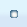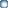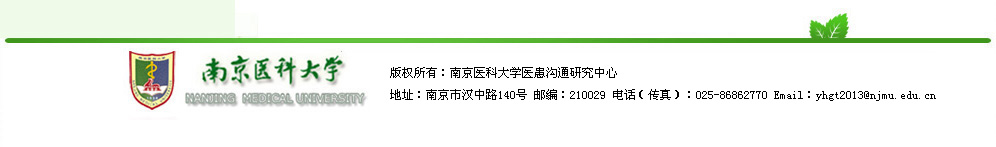国内研究国外研究
 当前位置:国内研究
  李峻,吴红梅,董碧蓉等.医学生与老年患者交流/沟通问题的调查分析[J].中国循证医学杂志,2009,9(1) 李钧,陈天葆.麻醉前谈话的方法和技巧[J].中国医学伦理学,2010,23(3)  范伟杰,徐雪梅.浅谈骨外科医患之间的沟通技巧[J].中国医学伦理学,2010,23(2) 林凯程,周梅芳.广州市综合型医院危机公关意识的现状调查与分析[J].中国医学伦理学,2010,23(3) 邵永祥.标准化病人教学方法在医患沟通实践教学中的探索[J].中国医学伦理学,2010,23(3) 周烁.关于医学生医患沟通教育的探讨[J].中国医学伦理学,2010,23(3) 黄正接,罗琪."同理心"在外科医患沟通中的应用[J].中国医学伦理学,2010,23(1) 李大畏,张大生,李晶尧.学导式创新体系康复中医患沟通理论与实践[J].按摩与康复医学（中旬刊）,2011,2(8) 徐金凤,张大生,李晶尧.重症康复中患者心理机制解析及医患沟通实践[J].按摩与康复医学（中旬刊）,2011,2(8) 刘新莉.儿童牙科术前沟通对患儿配合程度影响的临床研究[J].临床医学,2011,31(8) 白慧英.医患沟通与知情同意在医疗及病案管理中的作用[A].全国医院病案管理、统计工作新进展(重庆)学术研讨会论文集[C].2009. 李欣,余占海.口腔科老年患者的主要心理问题及接诊对策[J].中国中医药咨讯,2011,3(8) 张学英,王朝华,王光林.探讨医患纠纷的原因及防范措施[J].求医问药（学术版）,2011,9(8) 何伟.浅析医患沟通的方法与技巧[J].医药前沿,2011,01(8) 梁麒.提倡以人为本加强医患沟通[J].中外医学研究,2011,09(8) 陈道荣,王丕龙.如何提高实习医生的医患沟通能力[J].医学教育探索,2009,8(7) 毛怀东,宋海锋.综合医院ICU医患沟通重点环节及技巧[J].中国当代医药,2011,18(18) 徐宝君,张大生,李晶尧.关于传统医学非药物疗法医患沟通技巧的探讨[J].按摩与康复医学（下旬刊）,2011,2(7) 童志敏.手术室整体护理医患沟通情况调查分析[J].中国中医药咨讯,2011,3(18) 赵光斌,林敏,袁素平.当前医疗执业环境与门诊投诉的原因和特点分析[A].全国加强门诊急诊工作(大连)学术研讨会论文集[C].2009. 叶丽娜.北京市某三级甲等综合医院医师医患沟通态度、行为调查[J].中国医学创新,2011,08(18) 张国伟.浅析医患沟通在防范医疗纠纷中的作用及实践[J].卫生职业教育,2011,29(7) 沈红学.人文精神与医患和谐[J].中国美容医学,2010,19(z4) 邓燕京.剖析医患矛盾改善医患关系[J].中国美容医学,2010,19(z4) 刘同亭,薛源,朱建军等.美容外科医患沟通应注意的问题[J].中国美容医学,2010,19(z3) 沈红学.改善医患矛盾和谐医患关系[J].中国美容医学,2010,19(z3) 宋金德.社区健康服务中心构建和谐医患关系的看法[J].中国美容医学,2010,19(z2) 潘承恩.做一个称职的外科医生[J].中国医学伦理学,2010,23(1) 王仲.掌握医患沟通技巧，防范医疗风险[A].第四届中国肿瘤内科大会论文集[C].2010. 杜生华.论医患纠纷及其对策[J].中国社会医学杂志,2010,27(1) 王雁,王艾.加强医患沟通构建和谐医患关系[J].中国医院,2009,13(9) 吴亮君,罗天友,陈鸿雁等.加强实习医师医患沟通能力培养的探讨[J].医学教育探索,2009,8(10) 陈建斌.加强临床实习医学生医患沟通能力的培养[J].医学教育探索,2009,8(1) 周生明.知情沟通与医患关系[J].中国实用乡村医生杂志,2011,18(6) 马秋芳.医患沟通在构建和谐医患关系中的作用[J].临床合理用药杂志,2011,04(17) 郑恩同,郑力.医患沟通的新途径:医疗纠纷听证会制度[J].中国医院,2009,13(9) 李林波.医患沟通是构建和谐医患关系的有效途径[J].中国实用乡村医生杂志,2011,18(5) 方全明.沟通无处不在,沟通从心开始——开展“沟通月”活动的体会[A].中华医学会全科医学分会第六届学术年会论文集[C].2008. 贺艳.医患沟通在妇产科工作中的重要性[J].按摩与康复医学（下旬刊）,2011,02(5) 李新建.肾活检穿刺术前后医患沟通与观察[J].中外健康文摘,2011,08(16) 古文珍.口腔门诊医患沟通量表的研制及沟通干预方法的研究[D].中山大学,2009. 周淑芳.新时期构建和谐医患关系的若干思考[J].中国社区医师（医学专业）,2011,13(16) 贾佩娟.浅谈医患沟通中医用语言的语用规范[J].中国中医药咨讯,2011,03(5) 丁四清,谢建飞,易琦峰.新形势下和谐医患关系的构建[J].当代护士（学术版）,2011,(5) 钟碧.影响妇产科临床教学的原因及相应对策[J].健康必读（下旬刊）,2011,(4) 高秀娥.浅谈医患关系及其在儿科的临床实践[J].求医问药（学术版）,2011,9(4) 翟程花.加强医患沟通搞好医患关系[J].中外健康文摘,2011,08(15) 刘庆龙.医患沟通在处理医患矛盾中的应用[A].中华中医药学会2008年学术年会论文集[C].2008. 阿米纳·吐尔逊.口腔医疗过程中的医患沟通[J].中国中医药咨讯,2011,03(15) 黄洁明,罗颂平,廖雅琪.加强中西医结合学生医患沟通能力培训的探讨[J].中国中医药现代远程教育,2011,09(4) 周守君,顾慧,安媛媛.肿瘤病人医疗服务中的医患沟通路径研究[J].中国医院管理,2007,27(7) 殷大奎.人文医学精神与医师职业责任[J].中国医学伦理学,2009,22(2) 卢佼佼.论新形势下医学生职业素质的培养[J].中医药管理杂志,2011,19(3) 韩永祥,胡敏,汪红兵.基层中医药人才继续教育及对策思考[J].中医药管理杂志,2011,19(3) 黄启武.新时期构建和谐医患关系的思考[J].都市家教(下半月),2011,(3) 白宏治,张银峰.加强医患沟通及减少医疗纠纷的对策[J].中国当代医药,2011,18(14) 胡志芳.浅谈医患沟通[A].中华中医药学会2008年学术年会论文集[C].2008. 王天栋.浅议全科医生如何在交流中建立和谐的医患关系[J].亚太传统医药,2011,07(3) 林一民.门诊检验医患沟通技巧探讨[J].重庆医学,2007,36(11) 戴晓婧,谢峻,王琳.我国医患沟通的不良表现及对策[J].解放军医院管理杂志,2009,16(7) 王静.提高患者满意度构建和谐医患关系[J].中国现代医生,2011,49(13) 丁四清,谢建飞,熊灿娟.医患沟通的实践[J].中外医疗,2011,30(13) 侯云华.加强医护患间沟通对提高手术护理安全的作用[J].中外健康文摘,2011,8(25) 王伟.如何加强医患沟通[J].临床合理用药杂志,2011,4(25) 徐科.院前急救的医患沟通技巧[J].中国社区医师（医学专业）,2011,13(25) 张惠红.老年痴呆患者的沟通技巧[A].2008年天坛国际脑血管病会议论文集[C].2008. 沈凌云.老年病房医患沟通的做法及成效[J].中国老年保健医学,2011,09(2) 李春年,李淑娟,杨冬茹等.临床教学中医患沟通技巧培养的体会[J].继续医学教育,2011,25(2) 范兵.28例肋骨骨折X线检查漏诊原因分析及医患关系的处理对策[J].中国社区医师（医学专业）,2011,13(13) 陈英云,王艳萍.加强医患沟通减少医患纠纷[J].中国医院管理,2006,26(7) 王光明,董耀斌,王朝霞等.医患沟通在医疗活动中的作用与意义[J].中国卫生质量管理,2009,16(5) 李斌,孙晓阳,王锦帆.医患沟通障碍因素研究综述[J].中国卫生事业管理,2009,26(5) 马涵英,周玉杰,张维君.研究生医患沟通素质培养的探讨[J].卫生职业教育,2010,28(12) 朱春晖.临床教学中提高实习医生医患沟通能力的探讨[J].内科,2011,06(1) 黄宏敏.加强医患沟通防范医疗纠纷方法的运用与探讨[A].2008年全国静脉治疗护理新进展论坛论文集[C].2008. 黄颖,张春强,黎承平等.加强血液科临床实习生医患沟通能力培养的探索[J].西北医学教育,2011,19(1) 吉俊翠.浅谈医患沟通[J].中国实用乡村医生杂志,2011,18(1) 陈小琴,焦海燕,梁自芬.医疗活动中医患沟通的地位[J].中国当代医药,2011,18(1) 王晓桃.广州市某大型综合性医院医患沟通现状调查[D].中山大学,2009. 施根林,丁天鹏.《医患沟通制度》实施前后医患沟通情况调查分析[J].武警医学,2009,20(7) 薛春雨,王志农,邢新.标准化病人在医学生医患沟通技能培训中的应用[J].中国医学教育技术,2009,23(3) 李晓光,刘娜.临床实习医师医患沟通技能培养[J].解放军医院管理杂志,2008,15(11) 王烜,夏国栋,邓明明.医患沟通——医学生必备的素质[J].中国医学伦理学,2009,22(5) 任智红.论有效医患沟通的基础条件[J].管理观察,2010,(35) 李静,王江滨,王丽范.建立ICU护患沟通制度预防护理纠纷[A].中华医学会2008年全国重症医学研讨会论文集[C].2008. 池红梅,陈钰仁,何剑.谈和谐医患关系建设中医患沟通的意义[J].中国医药导报,2010,07(23) 孙治国.医患沟通的重要性[J].中国社区医师（医学专业）,2010,12(23) 高云.探讨医患沟通在洁治术中实践的意义[J].中外医学研究,2010,8(12) 赵茜,赵亮.医患沟通行为及其规范化管理探讨[J].中国医学伦理学,2009,22(6) 尚湘原.刍议非语言性沟通与和谐医患关系的构建[J].中国医学伦理学,2009,22(2) 李广清,顾勤,王沛等.开设医患沟通实训课程的基本思路[J].中外医疗,2010,29(34) 邢红.论新时期和谐医患关系的构建及对策研究[J].亚太传统医药,2010,06(11) 蒋步锦.医患沟通现状分析及探讨[J].中国医药导报,2010,07(34) 林兵英.以人为本建立信任、尊重、良好的医患关系[A].2009年医院管理论坛年会论文集[C].2009. 侯素青,王国安.加强医患沟通管理提升医疗服务满意度[J].中医药管理杂志,2010,18(11) 姚丹.护理在医患沟通中的作用[J].医学信息（中旬刊）,2010,05(11) 方劲松.如何构建和谐医患关系[J].医学理论与实践,2010,23(11) 王丽娟.医疗服务中医患沟通的意义和方法[J].中国社区医师（医学专业）,2010,12(22) 黄明慧.从糖尿病患者就诊经历浅析医患沟通[J].中国医学创新,2010,07(22) 张茂莎,周莜璇,李林玲.巧妙而有效的医患沟通是防范医疗纠纷的关键[J].中国民族民间医药,2010,19(11)